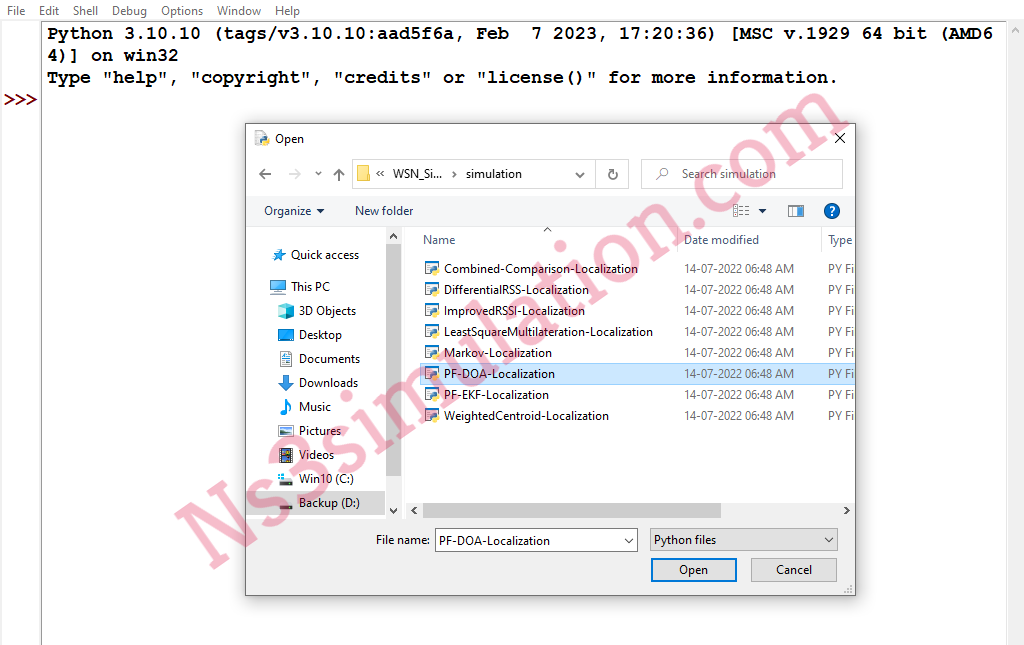Ns3 Projects for B.E/B.Tech M.E/M.Tech PhD Scholars.  Phone-Number:9790238391   E-mail: ns3simulation@gmail.com

# Can I Simulate Wireless Sensor Networks in Python If Yes Can You Provide the Links

Of course, each and every one of us can simulate the wireless sensor networks in python through following all the guidelines highlighted by our research professionals.

Stage: I

As the first process, we have to open the WSN in python IDE through going to the file and opening it using python to simulate the wireless sensor networks in python.Stage: II

While opening the WSN, the below mentioned python source code will be occurred for the sensor node localization.

``` import math import numpy as np import numpy as np import matplotlib.pyplot as plt from mpl_toolkits.mplot3d import Axes3D from matplotlib import pyplot as pb import random from datetime import datetime import time def dist(x, y, pos): return math.sqrt((pos-x)**2 + (pos-y)**2) areaSize=(30, 30) node_positions = (areaSize+6,areaSize+6) node_pos=[(-node_positions,node_positions),(node_positions,node_positions),(node_positions,-node_positions),(-node_positions,-node_positions)] initial_pos=(0,0) possible_value = list(range(-20, 20)) num_particles = 200 NOISE_LEVEL=1 RESOLUTION=5 STEP_SIZE=1/RESOLUTION def gen_wifi(freq=2.4, power=20, trans_gain=0, recv_gain=0, size=areaSize, pos=(5,5), shadow_dev=2, n=3,noise=NOISE_LEVEL): if pos is None: pos = (random.randrange(size), random.randrange(size)) random.seed(datetime.now()) rss0 = power + trans_gain + recv_gain + 20 * math.log10(3 / (4 * math.pi * freq * 10)) rss0=rss0-noise*random.random() normal_dist = np.random.normal(0, shadow_dev, size=[size+1, size+1]) rss = [] random.seed(datetime.now()) for x in range(0,4): distance = dist(node_pos[x], node_pos[x], pos) val =rss0 - 10 * n * math.log10(distance) + normal_dist[int(pos)][int(pos)] rss.append(val-noise*random.random()) return rss text=[] overall_rss=[] original_tragectory=[] Previous_pos = initial_pos ```

Stage: III

Finally, we have to simulate the WSN in python through going to the menu and run the module option as mentioned in the following image.In addition, we have highlighted the acquired results through the simulating the sensor positioning in WSN using python.If you have any issues while simulating WSN by your own then reach us and acquire the finest guidance.